# International Economics Study Set 8

## Quiz 13 : Exchange Rates and the Foreign Exchange Market: an Asset ApproachLooking for Economics Homework Help?

## Quiz 13 :Exchange Rates and the Foreign Exchange Market: an Asset Approach

Question TypeIn Munich, a bratwurst costs 5 euros; a hot dog costs $4 at Boston's Fenway Park. At an exchange rate of$1.05/per euro, what is the price of a bratwurst in terms of a hot dog? All else equal, how does this relative price change if the dollar depreciates to $1.25 per euro? Compared with the initial situation, has a hot dog become more or less expensive relative to a bratwurst? Free Essay Answer: Answer: Price of one bratwurst is 5euros and the price of hot dog is$4
Exchange rate = $1.05/per euro So, price of one bratwurst = 5 euros ×$1.05
= $5.25 Since the price of both bratwurst and hotdog is expressed in dollar terms, the price of bratwurst in terms of a hot dog =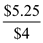= 1.3125 So one bratwurst is equal to 1.3 hotdogs, Therefore,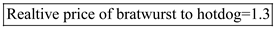Now, when the exchange rate is$1.25/per euro
Price of one bratwurst = 5 euros ×$1.25 =$6.25
The price of bratwurst in terms of a hot dog =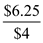= 1.5625
Therefore, one bratwurst is equal to 1.6 hotdogs,
Therefore,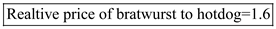With the change in exchange rate, that is dollar depreciates, each bratwurst is fetching more hotdogs implying that hot dog become cheaper than before.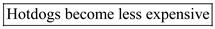TagsAs defined in footnote 3, cross exchange rates are exchange rates quoted against currencies other than the U.S. dollar. If you return to Table, you will notice that it lists not only exchange rates against the dollar, but also cross rates against the euro and the pound sterling. The fact that we can derive the Swiss franc/Israeli shekel exchange rate, say, from the dollar/franc rate and the dollar/shekel rate follows from ruling out a potentially profitable arbitrage strategy known as triangular arbitrage. As an example, suppose the Swiss franc price of a shekel were below the Swiss franc price of a dollar times the dollar price of a shekel. Explain why, rather than buying shekels with dollars, it would be cheaper to buy Swiss francs with dollars and use the francs to buy the shekels. Thus, the hypothesized situation offers a riskless profit opportunity and therefore is not consistent with profit maximization. Exchange Rate Quotations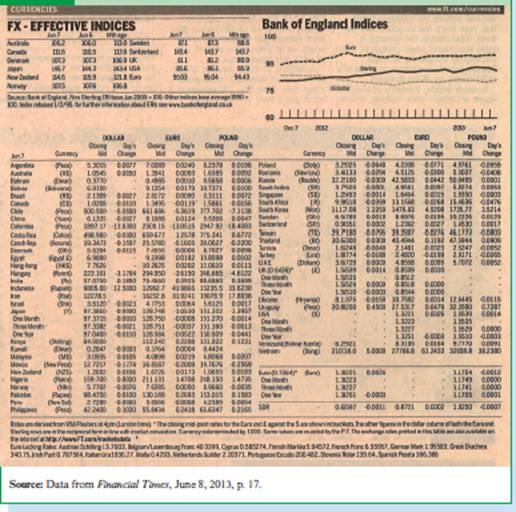Free
Essay

Suppose it is cheaper to purchase Swiss francs with dollars and later on exchange these francs with Israeli shekels than to directly purchase shekels with dollars. This will open a window for people to earn money through arbitraging.
The above scenario is characterized by an increase in the demand for Swiss francs from people who hold dollars. This would lead to a rise in the value of Swiss franc against the value of dollar.
This means that the Swiss franc will appreciate against the dollar until the price of a shekel would be exactly the same whether it was purchased directly with dollars or indirectly through Swiss francs.
For example, consider the exchange rate between the Swiss franc, the US dollar, and the Israeli shekels.
A dollar is worth 0.9351 Swiss francs, while a Swiss franc is worth 3.8595 shekels.
To rule out the triangular arbitrage, compare the value of exchange rates (direct and indirect))and find out which one gives a higher value of shekels.
Consider first, that Swiss francs are purchased with dollars (at an exchange rate of 0.9351 Swiss francs per dollar). Later on, these francs are used to buy Shekels.
In other words, compute the following case:Here, ' E ' stands for the exchange rate.
Hence, one dollar is worth 3.6090 shekels.
Therefore, in order to rule out triangular arbitrage, this indirect rate should exactly be equal to the direct exchange rate of shekels with dollars.
As the calculated value of shekels per dollar is greater than to the direct rate of shekels per dollar , there is a possibility of arbitrage.
The triangular arbitrage is " approximately " ruled out in above case for several reasons given as follows:
• First, rounding error means that there may be some small discrepancies between the direct and indirect exchange rates calculated above.
• Second, the transactions cost on trading currencies is a factor that will prevent the occurrence of arbitrage.

TagsTable reports exchange rates not only against the US dollar, but also against the euro and the pound sterling. (Each row gives the price of the dollar, euro, and pound, respectively, in terms of a different currency.) At the same time, the table gives the spot dollar prices of the euro ($1.3221 per euro) and the pound sterling ($1.5539 per pound). Pick any five currencies from the table and show that the three quoted spot exchange rates (in terms of dollars, euros, and pounds) approximately rule out triangular arbitrage. Why do we need to add the word "approximately"? Exchange Rate QuotationsFree
Essay

Triangular arbitrage is the process of converting one currency to another, converting it again to a third currency and, finally, converting it back to the original currency within a short time span. This opportunity for riskless profit arises when the currency's exchange rates do not exactly match up.
For example, take up Indian Rupees as one currency where we can rule out the possibility of triangular arbitrage. The data used is as follows:The arbitrage opportunity can be discussed as follows:There is a loss of Rupees 37 in the arbitrage which may be due to the transaction costs or taxes. Thus, the arbitrage here earns no profit or loss and the initial amount which was started with, returned back to approximately the same amount.
The word approximation is used here because, the calculation was done on real time data and a difference can be obtained because of daily fluctuations in the exchange rate or the inclusion of transaction costs or taxes put on arbitrage which are not known.

TagsPetroleum is sold in a world market and tends to be priced in U.S. dollars. The Nippon Steel Chemical Group of Japan must import petroleum to use in manufacturing plastics and other products. How are its profits affected when the yen depreciates against the dollar?
Essay
TagsCalculate the dollar rates of return on the following assets: a. A painting whose price rises from $200,000 to$250,000 in a year. b. A bottle of a rare Burgundy, Domaine de la Romanée-Conti 2011, whose price rises from $255 to$275 between 2013 and 2014. c. A £10,000 deposit in a London bank in a year when the interest rate on pounds is 10 percent and the $/£ exchange rate moves from$1.50 per pound to $1.38 per pound. Essay Answer: TagsWhat would be the real rates of return on the assets in the preceding question if the price changes described were accompanied by a simultaneous 10 percent increase in all dollar prices? Essay Answer: TagsSuppose the dollar interest rate and the pound sterling interest rate are the same, 5 percent per year. What is the relation between the current equilibrium$/£ exchange rate and its expected future level? Suppose the expected future $/£ exchange rate,$1.52 per pound, remains constant as Britain's interest rate rises to 10 percent per year. If the U.S. interest rate also remains constant, what is the new equilibrium $/£ exchange rate? Essay Answer: TagsTraders in asset markets suddenly learn that the interest rate on dollars will decline in the near future. Use the diagrammatic analysis of this chapter to determine the effect on the current dollar euro exchange rate, assuming current interest rates on dollar and euro deposits do not change. Essay Answer: TagsWe noted that we could have developed our diagrammatic analysis of foreign exchange market equilibrium from the perspective of Europe, with the euro / dollar exchange rate E €/$ (=1/ E $/€ ) on the vertical axis, a schedule vertical at R : to indicate the euro return on euro deposits, and a downward-sloping schedule showing how the euro return on dollar deposits varies with E €/$. Derive this alternative picture of equilibrium and use it to examine the effect of changes in interest rates and the expected future exchange rate. Do your answers agree with those we found earlier?
Essay
TagsThe following report appeared in the New York Times on August 7, 1989 ("Dollar's Strength a Surprise," p. D1): But now the sentiment is that the economy is heading for a "soft landing," with the economy slowing significantly and inflation subsiding, but without a recession. This outlook is good for the dollar for two reasons. A soft landing is not as disruptive as a recession, so the foreign investments that support the dollar are more likely to continue. Also, a soft landing would not force the Federal Reserve to push interest rates sharply lower to stimulate growth. Falling interest rates can put downward pressure on the dollar because they make investments in dollar-denominated securities less attractive to foreigners, prompting the selling of dollars. In addition, the optimism sparked by the expectation of a soft landing can even offset some of the pressure on the dollar from lower interest rates. a. Show how you would interpret the third paragraph of this report using this chapter's model of exchange rate determination. b. What additional factors in exchange rate determination might help you explain the second paragraph?
Essay
TagsSuppose the dollar exchange rates of the euro and the yen are equally variable. The euro, however, tends to depreciate unexpectedly against the dollar when the return on the rest of your wealth is unexpectedly high, while the yen tends to appreciate unexpectedly in the same circumstances. As a U.S. resident, which currency, the euro or the yen, would you consider riskier?
Essay
TagsDoes any of the discussion in this chapter lead you to believe that dollar deposits may have liquidity characteristics different from those of other currency deposits? If so, how would the differences affect the interest differential between, say, dollar and Mexican peso deposits? Do you have any guesses about how the liquidity of euro deposits may be changing over time?
Essay
TagsIn October 1979, the U.S. central bank (the Federal Reserve System) announced it would play a less active role in limiting fluctuations in dollar interest rates. After this new policy was put into effect, the dollar's exchange rates against foreign currencies became more volatile. Does our analysis of the foreign exchange market suggest any connection between these two events?
Essay
TagsImagine everyone in the world pays a tax of t percent on interest earnings and on any capital gains due to exchange rate changes. How would such a tax alter the analysis of the interest parity condition? How does your answer change if the tax applies to interest earnings but not to capital gains, which are untaxed?
Essay
TagsSuppose the one-year forward $/€ : exchange rate is$1.26 per euro and the spot exchange rate is \$1.2 per euro. What is the forward premium on euros (the forward discount on dollars)? What is the difference between the interest rate on one-year dollar deposits and that on one-year euro deposits (assuming no repayment risk)?
Essay
TagsEurope's single currency, the euro, was introduced in January 1999, replacing the currencies of 11 European Union members, including France, Germany, Italy, and Spain (but not Britain; see Chapter 10). Do you think that, immediately after the euro's introduction, the value of foreign exchange trading in euros was greater or less than the euro value of the pre-1999 trade in the 11 original national currencies? Explain your answer.
Essay
TagsMultinationals generally have production plants in a number of countries. Consequently, they can move production from expensive locations to cheaper ones in response to various economic developments-a phenomenon called outsourcing when a domestically based firm moves part of its production abroad. If the dollar depreciates, what would you expect to happen to outsourcing by American companies? Explain and provide an example.
Essay